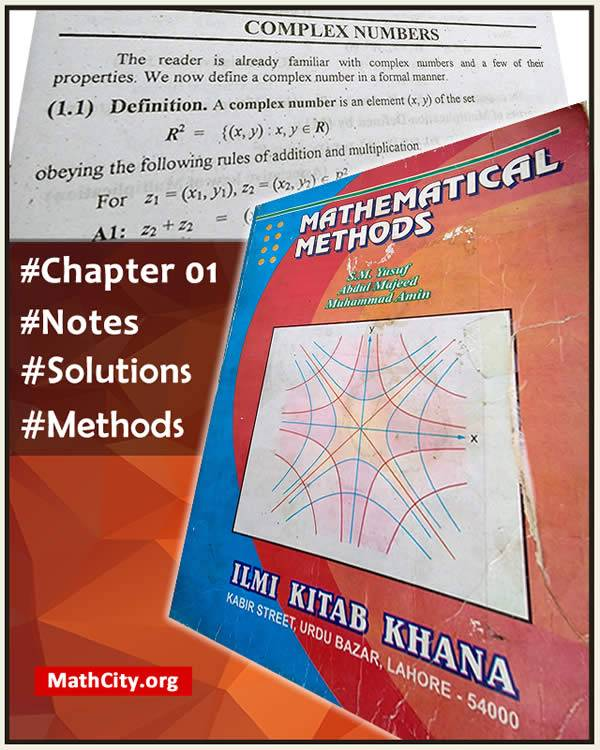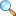# Chapter 01: Complex NumbersNotes of the book Mathematical Method written by S.M. Yusuf, A. Majeed and M. Amin, published by Ilmi Kitab Khana, Lahore - PAKISTAN.

A complex number is an element $(x,y)$ of the set $$\mathbb{R}^2=\{(x,y): x,y \in \mathbb{R}\}$$ obeying the following rules of addition and multiplication.

For $z_1=(x_1,y_1)$, $z_2=(x_2,y_2)$, we put

1. $z_1+z_2= (x_1+x_2, y_1+y_2)$
2. $z_1 z_2 = (x_1 x_2 - y_1 y_2, x_1 y_2+y_1 x_2)$

The set $\mathbb{R}^2$ with operation defined above is denoted by $\mathbb{C}$.

• Complex numbers
• Properties of complex numbers
• The Argand's diagram
• De Moivre's theorem
• Roots of the complex numbers
• Basic elementary functions
• Logarithmic functions
• Inverse hyperbolic functions
• Inverse trigonometric functions
• Complex power
• Summation of series
• Exercise 1.1 |View online | Download PDF (471KB)
• Exercise 1.2 |View online | Download PDF (1306KB)
• Exercise 1.3 |View online | Download PDF (1684KB)
• Exercise 1.4 |View online | Download PDF (654KB)
• Exercise 1.5 |View online | Download PDF (691KB)

#### Notes by Prof. M. Tanveer

• Exercise 1.1 |View online | Download PDF (715KB)• Exercise 1.2 |View online | Download PDF (2082KB)• Exercise 1.3 |View online | Download PDF (1332KB)• Exercise 1.4 |View online | Download PDF (1037KB)• Exercise 1.5 |View online | Download PDF (652KB)• bsc/notes_of_mathematical_method/ch01_complex_numbers